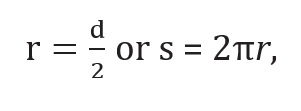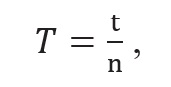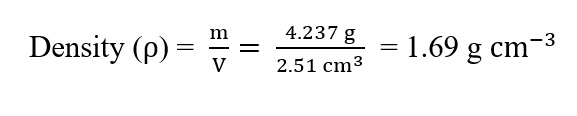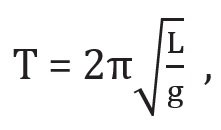Significant Figures: As discussed above, every measurement involves errors. Thus, the result of measurement should be reported in a way that indicates the precision of measurement. Normally, the reported result of measurement is a number that includes all digits in the number that are known reliably plus the first digit that is uncertain. The reliable digits plus the first uncertain digit are known as significant digits or significant figures.

A choice of change of different units does not change the number of significant digits or figures in a measurement.

For example, the length 2.308 cm has four significant figures. But in different units, the same value can be written as 0.02308 m or 23.08 mm or 23080 μm.

Rules:

• All the non-zero digits are significant.
• All the zeros between two non-zero digits are significant, no matter where the decimal point is, if at all.
• If the number is less than 1, the zero(s) on the right of decimal point but to the left of the first non-zero digit are not significant. [In 0.00 2308, the underlined zeroes are not significant].
• The terminal or trailing zero(s) in a number without a decimal point are not significant. [Thus 123 m = 12300 cm = 123000 mm has three significant figures, the trailing zero(s) being not significant.]
• The terminal or trailing zero(s) in a number with a decimal point are significant. [the numbers 2.500 or 0.06800 have four significant figures each.]
• For a number greater than 1, without any decimal, the trailing zero(s) are not significant.
• The digit 0 conventionally put on the left of a decimal for a number less than 1 (like 0.1250) is never significant. However, the zeroes at the end of such number are significant in a measurement.
• The multiplying or dividing factors which are neither rounded numbers nor numbers representing measured values are exact and have an infinite number of significant digits. For example infactor 2 is an exact number and it can be written as 2.0, 2.00 or 2.0000 as required. Similarly, inn is an exact number.

## Rules for Arithmetic Operations with Significant Figures

• In multiplication or division, the final result should retain as many significant figures as are there in the original number with the least significant figures.
For example, density should be reported to three significant figures.• In addition or subtraction, the final result should retain as many decimal places as are there in the number with the least decimal places.
For example,

436.32 g + 227.2 g + 0.301g = 663.821g (But the least precise measurement (227.2 g) is correct   to only one decimal place. The final result should, therefore, be rounded off to 663.8 g.)

Similarly, For Difference
0.307 m – 0.304 m = 0.003 m = 3 ×  m.

## Rounding off the Uncertain Digits

Rules:

• The preceding digit is raised by 1 if the insignificant digit to be dropped (the underlined digit in this case) is more than 5, and is left unchanged if the latter is less than 5.
• But what if the number is 2.745 in which the insignificant digit is 5. Here, the convention is that if the preceding digit is even, the insignificant digit is simply dropped and, if it is odd, the preceding digit is raised by
For example, the number 2.745 rounded off to three significant figures becomes 2.74. On the other hand, the number 2.735 rounded off to three significant figures becomes 2.74 since the preceding digit is odd.
• In any involved or complex multi-step calculation, you should retain, in intermediate steps, one digit more than the significant digits and round off to proper significant figures at the end of the calculation.
• A number known to be within many significant figures, such as in 2.99792458 × 108 m/s for the speed of light in vacuum, is rounded off to an approximate value 3 × 108 m/s, which is often employed in computations.
Remember that exact numbers that appear in formulae like 2π inhave a large (infinite) number of significant figures. The value of π = 3.1415926…. is known to a large number of significant figures. You may take the value as 3.142 or 3.14 for π, with a limited number of significant figures as required in specific cases.

## Rules for Determining the Uncertainty in the Results of Arithmetic Calculations:

1. If the length and breadth of a thin rectangular sheet are measured, using a metre scale as 16.2 cm and, 10.1 cm respectively, there are three significant figures in each measurement.
It means that the length l may be written as
l = 16.2 ± 0.1 cm
= 16.2 cm ± 0.6 %.
Similarly, the breadth b may be written as
b = 10.1 ± 0.1 cm
= 10.1 cm ± 1 %
Then, the error of the product of two (or more) experimental values, using the combination of errors rule,
will be
l b = 163.62  ± 1.6%
= 163.62 ± 2.6
This leads us to quote the final result as
l b = 164 ± 3
Here 3  is the uncertainty or error in the estimation of the area of the rectangular sheet.
• If a set of experimental data is specified to n significant figures, a result obtained by combining the data will also be valid to n significant figures.
• The relative error of a value of number specified to significant figures depends not only on n but also on the number itself.
• Intermediate results in a multi-step computation should be calculated to one more significant figure in every measurement than the number of digits in the least precise measurement.